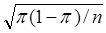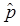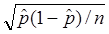Terms investigation Definition Addition rule for disjoint events Inv 0 The probability of the union of disjoint events (no shared outcomes) is the sum of the probabilities of the individual events. Alternative hypothesis Inv 1.2 A statement of the parameter values specified by the research conjecture. Inv 1.1 A graphical display of categorical data with a bar for each category.  The height of the bar indicates the frequency or the proportion of observations in that category.  Bars are typically the same width and with gaps between bars. Basic regression model Inv 4.12 The model assuming linearity between x and y, equal variance in responses at each x, and normality of the responses at each x. Inv 1.12 A sampling method that consistently overrepresents or underrepresents distinct segments of the population Binary variable Inv 1.2 A categorical variable with only two possible outcomes (e.g., heads, tails) Binomial Inv 1.1 Expl A probability distribution modeling the number of successes in a fixed number of independent trials with a constant probability of success. Boxplot Inv 3.1 A graphical display of the five number summary. The box extends from the lower quartile to the upper quartile with a vertical line at the median. Whiskers extend to min and max values or to the most extreme non-outlier values (using the 1.5IQR rule) Categorical variable Inv 1.2 A variable that places observational units into categories (e.g., small, medium, or large), rather than measuring a numerical value. Central Limit Theorem of the sample proportion Inv 1.7 The sampling distribution of a sample proportion is approximately normal for large sample sizes with mean equal to the population proportion/process probability and standard deviation equal to. Chi-squared distribution Inv 4.1 A right-skewed probability distribution that models the behavior of the chi-square statistic under the null hypothesis. Chi-squared test statistic Inv 4.1 A statistic summarizing the discrepancies between the observed counts in a two-way table and the expected counts under the null hypothesis. Coefficient of determination Inv 4.8 The percentage of variability in the response variable that is explained by the regression on the explanatory variable. Complement rule Inv 0 The probability of the complement of the event equals one minus the probability of the event. Conditional proportions Inv 2.1 Calculating separate proportions for each category of the explanatory variable Confidence interval Inv 1.5 A set of plausible values of the parameter based on the observed sample statistic. Inv 1.5 The long-run proportion of intervals that capture the parameter value. If the procedure is valid the observed coverage rate under repeated random sampling will match the stated confidence level. Confounding variable Inv 2.2 A variable that changes between the explanatory variable groups and potentially impacts the response variable. Control group Inv 2.5 A group in a comparative experimental study that receives no treatment or a placebo treatment. Convenience sample Inv 1.9 A sample selected from a population using the most readily available observational units or process; generally not considered representative of the population or process. Correlation coefficient Inv 4.7 A numerical measure of the linear association between two quantitative variables. Could have been distribution Inv 1.1 The distribution of a simulated sample of data, generated according to an assumed null model Inv 1.10 The multiplier of the standard error in a confidence interval corresponding to the nominal confidence level. Data transformation Inv 3.10 A function applied to data that rescales the variable, often changing the shape and spread of the distribution. Transformations can be useful for normalizing a distribution to allow use of normal-based methods or for linearizing bivariate data to allow use of regression models. Degrees of freedom Inv 3.3 A number related to the number of “independent” observations in the calculation of a statistic. It is used to index a particular member of a probability distribution family. Discrete random variable Inv 0 A random variable that can take on a finite number or a countable number of possible values. Dotplot Inv 1.1 A graphical display of quantitative data where each observational unit is represented by a dot above the horizontal axis. Duality Inv 1.5 The correspondence between a two-sided test of significance and a confidence interval. Inv 1.7 For any mound-shaped, symmetric distribution, approximately 68% of observations fall within one standard deviation of the mean, 95% within 2 standard deviations and 99.7% within three standard deviations. Expected count Inv 4.1 The expected number of observations in a cell of a two-way table, assuming independence between the row and column variables = (row total)x(column total)/Table total Expected value Inv 0 In a probability distribution, a weighted average of possible outcomes of a random variable, with weights determined by the probability (or density) of the outcome, representing the long-run average outcome of the random variable. Experiment study Inv 2.3 A study that actively imposes the explanatory variable (or “treatments”) on the observational (“experimental”) units. Explanatory variable Inv 2.2 The variable in a study that we believe may be explaining the variation/behavior of the response variable.  In an experiment, this is the variable manipulated by the researchers. Extrapolation Inv 4.8 Making predictions at explanatory variable values far outside the range used to derive the regression equation Fisher's Exact Test Inv 2.7 Fixes the marginal totals in a two-way table and uses the hypergeometric distribution to calculate the probability of at least as many successes in group A as observed in the actual research study. Five Number Summary Inv 3.1 The minimum, lower quartile, median, upper quartile, and maximum Histogram Inv 0 A graphical display of quantitative data that groups the values into bins and then displays bars for each bin with height equal to the frequency or relative frequency of the observations in that bin Hypergeometric distribution Inv 1.15 A probability distribution that models the probability of observing X successes being selected randomly in a sample of n objects from a population with M successes and N-M failures. Independent trials Inv 1.1 Prob Detour Random trials from a random process where the probability of success or failure on a trial does not depend on the outcomes of any other trials. Influential observation Inv 4.9 An observation whose removal substantially changes the association between two variables. Interquartile range Inv 3.1 The difference between the upper quartile (75th percentile) and the lower quartile (25th percentile); a measure of variability Least squares line Inv 4.8 The line that minimizes the sum of the squared residuals (aka regression line) Inv 1.5 The cut-off for the p-value that leads us to reject the null hypothesis.  The probability of a type I error. Inv 1.10 The half-with of a confidence interval; the value that is added to and subtracted from the value of the statistic to determine the endpoints of the confidence interval. Median Inv 3.1 A value such that at least 50% of the observations in the data set are smaller than that value and at least 50% of the observations in the data set are larger than that value. Mutually exclusive event Inv 1.1 Prob Det. Sets of outcomes of a random process that do not share any outcomes in common. Non-sampling error Inv 1.15 An error in the data collection process that is not related to how the sample was selected (e.g., poor question wording) Inv 1.7 A probability model for mound-shaped symmetric, continuous distributions.  Completely characterized by the mean and standard deviation.  Probabilities correspond to areas under the curve; typically found using technology. Null distribution Inv 1.1 A distribution of statistics where the statistics have been randomly generated based on an assumed chance model Inv 1.1 A statement of the parameter values specified by the null model., typically representing "no effect" or "no difference" Null model Inv 1.1 A chance model associated with a null hypothesis.  Usually the “by chance alone” model. Observational study Inv 2.3 A study in which no variables are manipulated by the researchers. Instead data is recorded as it occurs naturally. Observational units Inv 1.2 The people or objects about which data are recorded. Odds of success Inv 2.10 The ratio of the number of successes to the number of failures; equivalently the ratio of the probability of success to the probability of failure. Odds ratio Inv 2.10 The ratio of the odds of success between two groups. One-sample z interval Inv 1.10 For estimating a process probability or a population proportion:+ z*; valid when have at least 10 successes and at least 10 failures. Outlier Inv 3.1 An observation that does not follow the general pattern of the other observations, typically an extreme minimum or maximum value. One way to "test" for outliers is identifying any observations that fall more than 1.5 × IQR from the nearest quartile as outliers. Inv 3.12 A confidence interval for the mean difference in response from a paired study design. Inv 3.12 A test of the mean of the differences in response in a paired study. Inv 1.2 A numerical summary describing the larger process than generated the data or to the population from which the sample was selected. Placebo effect Inv 2.5 The potential effect on the response variable of the power of suggestions (e.g., patients feeling better because they are told they are receiving medicine to help them feel better). Inv 1.1 A believable or reasonable claim, often about a parameter value. For example, a null model that is not rejected because the result of the study is not surprising under the null model. Pooled t-test Inv 3.8 A t-test for comparing two means assuming the two population standard deviations are equal and using the pooled estimate of the standard deviation in the standard error calculation Inv 1.12 The entire collection of observational units we are interested in. Power Inv 1.6 The probability of rejecting the null hypothesis at a particular alternative value of the parameter Inv 1.18 The consideration of whether an “effect” has meaning in a practical sense, given the context and the magnitude of the effect Prediction interval Inv 3.4 A confidence interval for individual (future) observations (rather than the population mean) Probability Inv 0 Long-run proportion of times that an event occurs when its random process is repeated indefinitely Process Inv 0 See random process:  A sequence of outcomes generated under identical conditions, usually with outcomes that cannot be perfectly predicted in advance. p-value Inv 1.1 Probability that a random process alone would produce a statistic as (or more) extreme as the observed statistical in the actual study Quantitative variable Inv 1.2 A variable that takes on numerical characteristics (where it makes sense to average the values of the outcomes) Random assignment Inv 2.4 Assigning experimental units to treatments at random, each unit is equally likely to receive each of the treatments; goal is to create treatment groups that are balanced on all potential confounding variables. Inv 0 A sequence of outcomes generated under identical conditions, usually with outcomes that cannot be perfectly predicted in advance. Random variable Inv 0 A variable that assigns numbers to outcomes from a random process.  For example, X = number of heads in 5 tosses of a fair coin. Randomized experiment Inv 2.5 A study in which the  researchers decide, using random assignment, which explanatory variable group each experiment unit will be in. Regression line Inv 4.8 Inv 1.6 The values of the statistic that lead us to reject the null hypothesis for a particular level of significance Relative risk Inv 2.9 The ratio of the conditional proportions of successes between two groups. Residual Inv 4.8 The “prediction error” between the observed result and the predicted result Resistant Inv 3.1 A numerical summary that t is not strongly affected by extreme observations (e.g., the median is a resistant measure of center) Response variable Inv 2.2 In a study, the variable that we think of as being explained by the explanatory variable.  In an experiment, this is the outcome variable of interest. Inv 1.12 The observational units for which we obtain measurements, a subset of the observational units in the population. Inv 1.2 The number of observational units in the study (for which data have been recorded). Typically denoted by n. Inv 1.12 An enumerated list of every member of the population used to select the sample. Sample space Inv 0 The list of all possible outcomes of a random process Inv 1.12 The property that the value of a statistic will vary from sample to sample but with a predictable pattern. Scatterplot Inv 4.6 A graphical display of the association between two quantitative variables. Segmented bar graph Inv 2.1 A graph for displaying a categorical response variable, with a separate bar for each category of the explanatory variable. Sign test Ex 3.4 A test of significance using the binomial distribution to count the number of quantitative values above a certain number (e.g., number of positive differences in paired study). Inv 1.12 A sampling method that gives every sample of size n an equal chance of being the selected sample. Simulation Inv 0 Artificially re-creating the outcomes of a random process, often using technology. Standard deviation Inv 1.7 The square root of the variance; a measure of spread in the outcomes of a distribution or random variable; roughly the average deviation from the mean of the distribution. Inv 1.10 An estimate of the standard deviation of a statistic based on sample data. Inv 1.8 Calculates the number of standard deviations an observation lies from the mean of the distribution. Inv 1.1 A numerical summary of a sample of data. Common examples are the sample proportion (categorical data) or the sample mean (quantitative data) Inv 1.1 An observed result that is found to be unlikely to happen by chance alone under the null model (small p-value). Inv 1.9 A measure of the discrepancy between the observed statistic and the parameter value(s) specified by the null hypothesis Inv 2.1 A test/interval comparing two sample proportions using the normal approximation Inv 1.4 A significance test for which no particular direction is specified in the alternative hypothesis, using "not equal to" in the alternative hypothesis. Two-way table Inv 2.1 A summary of counts cross-referenced by two categorical variables. Typically the explanatory variable is used as the column variable. Inv 1.6 Rejecting the null hypothesis when it is true. Inv 1.6 Failing to reject the null hypothesis when it is false. Unbiased sampling method Inv 1.12 A sampling method for which the generated statistics average out to the population parameter of interest. Inv 1.2 Any characteristic that varies from observational unit to observational unit Variance of random variable Inv 0 A weighted average of the squared deviations from the outcomes of the random variable and the expected value. What if distribution Inv 1.1 A distribution of statistics where the statistics have been generated according to an assumed null model. Inv 1.8 Calculates the number of standard deviations that an observation lies from the mean of the distribution.